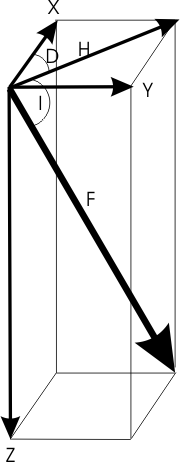# Magnetic components

The Earth's magnetic field is a vector quantity; at each point in space it has a strength and a direction. To completely describe it we need three quantities. These may be:

• three orthogonal strength components (X, Y, and Z);
• the total field strength and two angles (F, D, I); or
• two strength components and an angle (H, Z, D)

The relationship between these 7 elements is shown in the diagram.Magnetic components
Component Description
F the total intensity of the magnetic field vector
H the horizontal intensity of the magnetic field vector
Z the vertical component of the magnetic field vector; by convention Z is positive downward
X the north component of the magnetic field; X is positive northward
Y the east component of the magnetic field; Y is positive eastward
D magnetic declination, defined as the angle between true north (geographic north) and the magnetic north (the horizontal component of the field). D is positive eastward of true North.
I magnetic inclination, defined as the angle measured from the horizontal plane to the magnetic field vector; downward is positive

D and I are measured in degrees. All other elements are measured in nanotesla (nT; 1 nT = 10-9 Tesla).

The seven elements are related through the following simple expressions.

$\begin{array}{cc}\text{Declination (D)}& D={\mathrm{tan}}^{-1}\left(\frac{Y}{X}\right)\\ \text{Inclination (I)}& I={\mathrm{tan}}^{-1}\left(\frac{Z}{H}\right)\\ \text{Horizontal (H)}& H=\sqrt{{X}^{2}+{Y}^{2}}\\ \text{North (X)}& X=H\mathrm{cos}\left(D\right)\\ \text{East (Y)}& Y=H\mathrm{sin}\left(D\right)\\ \text{Intensity (F)}& F=\sqrt{{X}^{2}+{Y}^{2}+{Z}^{2}}\end{array}$

Use the magnetic field calculator to calculate the magnetic elements for any location.

Date modified: